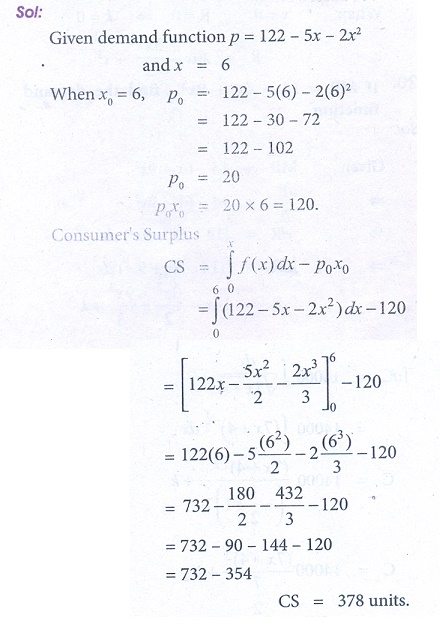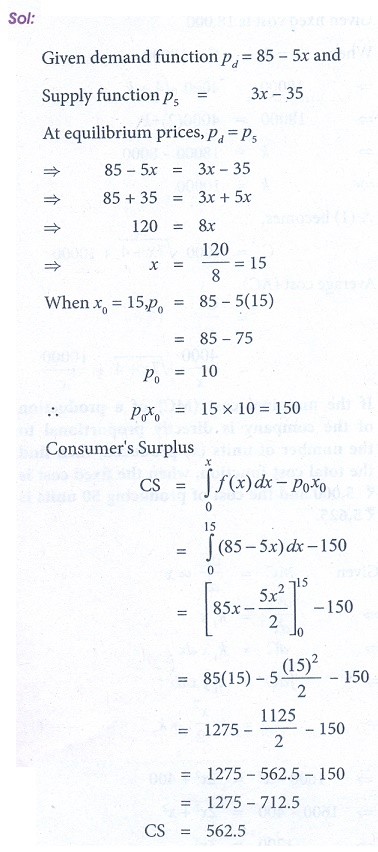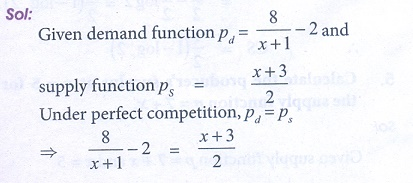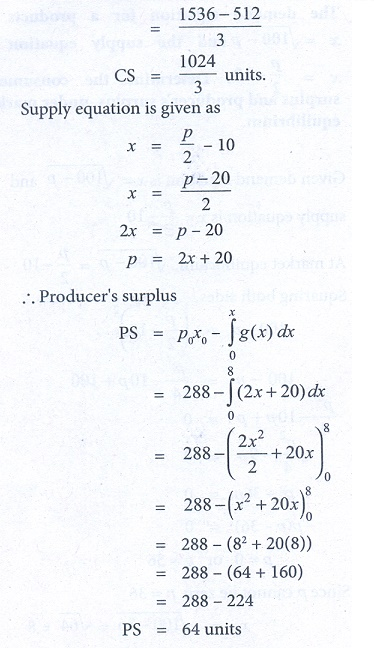Home | | Business Maths 12th Std | Exercise 3.3: Application of Integration in Economics and Commerce

# Exercise 3.3: Application of Integration in Economics and Commerce

Book back answers and solution for Exercise questions - Maths: Integral Calculus: Application of Integration in Economics and Commerce

Exercise 3.3

1. Calculate consumerŌĆÖs surplus if the demand function p = 50 ŌłÆ 2x and x = 202. Calculate consumerŌĆÖs surplus if the demand function p = 122 ŌłÆ 5x ŌłÆ 2x2 and x = 63. The demand function p = 85 ŌłÆ 5x and supply function p = 3x ŌłÆ 35 . Calculate the equilibrium price and quantity demanded .Also calculate consumerŌĆÖs surplus.4. The demand function for a commodity is p = eŌłÆx . Find the consumerŌĆÖs surplus when = 0.5.5. Calculate the producerŌĆÖs surplus at x = 5 for the supply function p = 7 + x.6. If the supply function for a product is p = 3x + 5x2 .Find the producerŌĆÖs surplus when = 4.7. The demand function for a commodity is p = 36/ x+4  . Find the consumerŌĆÖs surplus when the prevailing market price is Ōé╣6.8. The demand and supply functions under perfect competition are pd = 1600 ŌłÆ x2 and ps = 2x2 + 400 respectively. Find the producerŌĆÖs surplus.9. Under perfect competition for a commodity the demand and supply laws are pd = (8 / [x + 1]) ŌłÆ 2 and ps = [x + 3] / 2 respectively. Find the consumerŌĆÖs and producerŌĆÖs surplus.10. The demand equation for a products is x = ŌłÜ[100 ŌĆō p] and the supply equation is x = p/2 ŌłÆ10. Determine the consumerŌĆÖs surplus and producerŌĆÖs surplus, under market equilibrium.11. Find the consumerŌĆÖs surplus and producerŌĆÖs surplus for the demand function pd = 25 ŌłÆ 3and supply function ps = 5 + 2x.